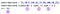Part of my series of easy primers on methods to eliminate code smell: needlessly lengthy or complex code.

## inject

Code smell: You’re performing a mathematical function on a series of numbers (like adding or multiplying) and returning the result.

This is a method with a very specific purpose, but when the time is right, it’s almost as satisfying as #each_with_object. That’s because the two iterators are very similar — but while #each_with_object adds elements to an array or hash, #inject just adds elements together. Or subtracts them, or multiplies them, etc.

#Inject’s uses are very straightforward, so let’s jump right in. Say you have an array of numbers and you want to sum them up. Without #inject, it would look like this:

With #inject, we can combine the steps of creating, incrementing and returning our total in a single step. Let’s take a look:1) in parentheses, we give a starting number for our mathematical operation. this argument is optional — without it, the method will just start with the first two numbers in the array (e.g. 5+10) 2) our first variable represents the accumulator object we want to return (a sum of the contributions), and our 2nd variable represents each number in our array 3) the mathematical operation we want to perform on our accumulator 4) #inject returns our sum

We call #inject on the array of numbers we want to combine. This method takes an optional argument: a starting number. If you don’t give a starting number, #inject will perform the operation on the first two numbers in your array. For example, if we were multiplying, we could either provide an initial argument of 1 (1 * 5 * 10 * 5…), or skip the argument, and #inject will perform (5 * 10 * 5…). Within our block, we give the mathematical operation we want to perform. Note that, unlike in a regular #each loop, we don’t have to specify sum += n (though we could). Here, the sum automatically gets passed through each iteration into the next one. At the end, #inject returns our total.

If we want, we can also pass in an existing value as our accumulator. For example, say we have the total of all our contributions, and now we have an array of expenses we want to subtract from it. With #inject, we can type:

Our second #inject method takes the total of our contributions as a starting point, and then subtracts from it each number in the expenses array. It returns our balances, which we can round down to 2 decimal places.

This method, as I mentioned above, has a very narrow field of use: repetitive mathematical operations on a series of numbers. But, when you’re in a position to do one of those, it’s a very useful method to cut down the length of your code.

Enjoy inject!

Written by

Written by

## Anna Rasshivkina

#### All things language. Writer, translator, coder. www.thesewords.live